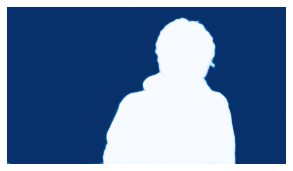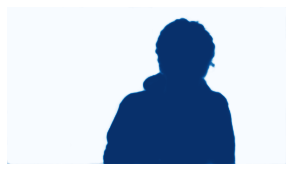In Part 1 we created our own dataset of webcam pictures and trained a model that separates the person from the background. Now, we're going to use this model to blur the background of a webcam video.

The Learner expects to find all functions that were defined when creating it, in our case that is create_mask. We don't need any custom functionality however, so we define an empty create_mask function.

def create_mask(): pass


Load the Learner we exported in Part 1. If you have not trained a model in part 1, you can download my model and play around. I can't guarantee that it works under any conditions other than my living room though 😀

learn = load_learner('unet-resnet18-person-background.pkl')


## Practicing Predictions

Note: You can skip this part and jump to the OpenCV part. I included this section because I wanted to see and show the different outputs of the predict function.

Let's pick a random file from our training images to practice getting the model predictions:

fnames = get_image_files('training')
image = fnames

PILImage.create(image).show();Get predictions of one training image:

preds = learn.predict(image)

preds


(TensorMask([[0, 0, 0,  ..., 0, 0, 0],
[0, 0, 0,  ..., 0, 0, 0],
[0, 0, 0,  ..., 0, 0, 0],
...,
[0, 0, 0,  ..., 0, 0, 0],
[0, 0, 0,  ..., 0, 0, 0],
[0, 0, 0,  ..., 0, 0, 0]]),
TensorImage([[0, 0, 0,  ..., 0, 0, 0],
[0, 0, 0,  ..., 0, 0, 0],
[0, 0, 0,  ..., 0, 0, 0],
...,
[0, 0, 0,  ..., 0, 0, 0],
[0, 0, 0,  ..., 0, 0, 0],
[0, 0, 0,  ..., 0, 0, 0]]),
TensorImage([[[9.9910e-01, 9.9987e-01, 9.9999e-01,  ..., 9.9790e-01,
9.8746e-01, 9.4114e-01],
[9.9981e-01, 9.9999e-01, 1.0000e+00,  ..., 9.9982e-01,
9.9790e-01, 9.7789e-01],
[9.9979e-01, 1.0000e+00, 1.0000e+00,  ..., 9.9998e-01,
9.9973e-01, 9.9559e-01],
...,
[7.8446e-01, 8.4830e-01, 8.4362e-01,  ..., 9.9416e-01,
9.8244e-01, 9.3761e-01],
[7.0075e-01, 7.4211e-01, 7.1380e-01,  ..., 9.8295e-01,
9.6359e-01, 9.0397e-01],
[6.7494e-01, 7.0632e-01, 6.6419e-01,  ..., 9.5549e-01,
9.1026e-01, 8.5593e-01]],

[[8.9632e-04, 1.3135e-04, 1.4032e-05,  ..., 2.0971e-03,
1.2543e-02, 5.8863e-02],
[1.8581e-04, 5.8454e-06, 2.6254e-07,  ..., 1.7936e-04,
2.1001e-03, 2.2113e-02],
[2.0952e-04, 4.2351e-06, 1.6508e-07,  ..., 1.7147e-05,
2.7214e-04, 4.4116e-03],
...,
[2.1554e-01, 1.5170e-01, 1.5638e-01,  ..., 5.8448e-03,
1.7561e-02, 6.2389e-02],
[2.9925e-01, 2.5789e-01, 2.8620e-01,  ..., 1.7050e-02,
3.6413e-02, 9.6029e-02],
[3.2506e-01, 2.9368e-01, 3.3581e-01,  ..., 4.4511e-02,
8.9740e-02, 1.4407e-01]]]))

There are different tensors in the predictions. preds contains the output after argmax, so it picks the class with the higher probability. Every pixel is either a 0 or a 1 in line with our two classes.

preds.show(cmap='Blues', vmin=0, vmax=1);print(f'''unique values: {np.unique(preds)}
type: {type(preds)}
data type: {preds.dtype}''')

unique values: [0 1]
data type: torch.int64


preds contains the same values, just in a different type (TensorImage instead of TensorMask)

preds.show(cmap='Blues', vmin=0, vmax=1);print(f'''unique values: {np.unique(preds)}
type: {type(preds)}
data type: {preds.dtype}''')

unique values: [0 1]
type: <class 'fastai.torch_core.TensorImage'>
data type: torch.int64


preds is a tensor with three dimensions. It contains the probabilities of the two classes as float values.

preds.shape

(2, 360, 640)

print(f'''unique values: {np.unique(preds)}
type: {type(preds)}
data type: {preds.dtype}''')

unique values: [4.5733633e-14 4.8081161e-14 5.0750907e-14 ... 9.9999988e-01 9.9999994e-01
1.0000000e+00]
type: <class 'fastai.torch_core.TensorImage'>
data type: torch.float32


Probabilities for the background class:

preds.show(cmap='Blues');Probabilities for the person class:

preds.show(cmap='Blues');## Constructing the Image With Blurred Background

We could use clean predictions preds with just 0s and 1s for a simple mask. I tried that initially and it worked, it resulted in some rough edges however.

Instead, we will use the raw probabilities from preds since it results in a smoother image. You can try for yourself which one you like btter.

Let's define a simple blur function.

def blur(img: np.ndarray, kernel_size=5, sigma_x=0) -> np.ndarray:
# Make sure that kernel size is an odd number
if kernel_size % 2 == 0:
kernel_size += 1
return cv2.GaussianBlur(img, (kernel_size, kernel_size), sigma_x)


We now define a function that blurs the background and blends in the original frame with an alpha mask. Thank you to learnopencv.com for their useful code!

def masked_blur(image: np.ndarray, mask: TensorImage) -> np.ndarray:
foreground = cv2.resize(image, (640,360), interpolation=cv2.INTER_AREA)
background = blur(foreground, kernel_size=61)

# Convert uint8 to float
foreground = foreground.astype(np.float32)
background = background.astype(np.float32)

# Some transforms to match the dimensions and type of the cv2 image

# Multiply the foreground with the alpha matte
foreground = cv2.multiply(alpha, foreground)
# Multiply the background with ( 1 - alpha )
background = cv2.multiply(1.0 - alpha, background)

# Convert to integer
result = result.astype(np.uint8)

return result


Read an image and create predictions:

frame = cv2.imread(str(image))
preds = learn.predict(image)
alpha = preds


Create the resulting image and have a look:

output = masked_blur(frame, alpha)
output_rgb = cv2.cvtColor(output, cv2.COLOR_BGR2RGB)

PILImage.create(output_rgb)Apart from my grumpy look, I think this is a quite nice result!

## Processing a Video Clip

As for now, we just work with a saved video file. To work with live webcam video, we would have to increase the speed of the inference process by a lot. On my current Paperspace Gradient machine (P4000) it runs at about 0.5 FPS....

Setting up video files. testclip.mp4 is a video I shot with my webcam. The arguments for the VideoWriter are framerate and dimensions. I chose 25 because I think this is the framerate of my webcam, and 640x360 are the dimensions we used to train the neural net.

cap = cv2.VideoCapture('testclip.mp4')
fourcc = cv2.VideoWriter_fourcc(*'mp4v')
out = cv2.VideoWriter('output/testclip-output.mp4', fourcc, 25, (640, 360))


### Main Loop

We use this while loop to capture every frame of the video. For every frame we

1. Resize it to 640x360
2. Convert it to from cv2 BGR to RGB
3. Use the model to predict the mask
4. Create the image with blurred background
5. Write this image to the output video

Additionally, we save some frames as jpg files to inspect them.

i = 0
while cap.isOpened():
# Capture frame

# Break loop at end of video
if ret == False:
break

# Resize frame and convert to RGB
frame = cv2.resize(frame, (640,360), interpolation=cv2.INTER_AREA)
frame_rgb = cv2.cvtColor(frame, cv2.COLOR_BGR2RGB)

# Run inference and create alpha mask from result
preds = learn.predict(frame_rgb)

# Blur background and convert it to integer type

# Write frame to video
out.write(output)

# Save every 25th output as jpg, just to find a good thumbnail :)
if i == 0 or i%25 == 0:
cv2.imwrite('output/output_'+str(i)+'.jpg', output)

# Increase counter
i += 1

# Release opened files
cap.release()
out.release()


### Results

Let's look at a single frame:

PILImage.create('output/output_0.jpg')And the resulting video:

I think that looks quite good. There are some rough edges and my arms are not recognized well, but overall I'm happy with the result for this little project.

## To Do

There are many aspects which we could improve:

• The biggest thing to improve now is inference speed. As I mentioned, the current implementation works only with video files, not live video, and it runs at about 0.5 frames per second 🥴

• The U-Net is a pretty heavy model, even with the relatively small Resnet18 backbone. The saved weights are 167MB. This alone is reason enough for the model to run slow. Since we run the model frame by frame, the GPU is not helping much because there is no parallelization.

• The next step would be better generalization. I suspect that this model is currently very much optimized for myself. If we wanted to roll this out as a feature for many people, we would have to include many people in our training dataset, as well as different backgrounds, cameras, and lightning situations.

• Aesthetics could be improved. There is a "shadow" around the person in the foreground, an artifact of blurring the whole picture including the person.

Let me know when you found this helpful or implemented something similar yourself, or if you're stuck. I'd be happy to hear from you on Twitter!